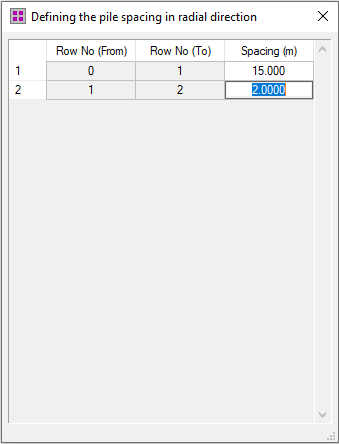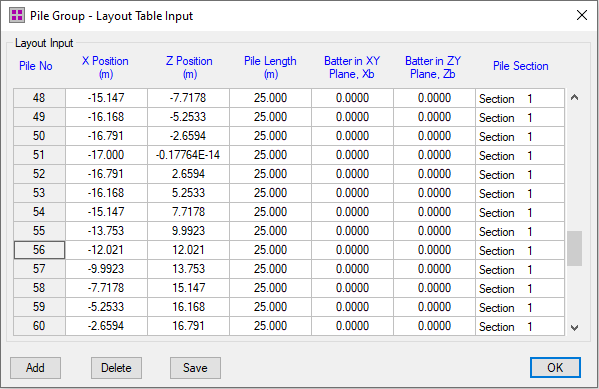top of page

# Circular pile group layout for wind turbine foundations in PileGroup program

Circular pile group layout can be generated easily for wind turbine foundations in the PileGroup program.

A pile length and layout wizard tool is provided by the program to quickly generate the pile group layout in either rectangular or circular shape. This tool is suitable for the case where the piles within the pile group have the regular spacings in both X and Z directions for either rectangular or circular grid layout. The pile length and the section type will be the same for each individual pile for the pile group created by this tool. If required, further refinement/adjustment can be undertaken for any pile within the pile group by the conventional dialog or table input method.

The following figure shows a typical dialog layout for defining the circular pile group layout. There are two main input parameters for the circular pile group layout: (1) Number of rows in radial direction and (2) Number of rows in Tangential direction.The spacing between piles in radial direction can be input by the users by clicking the "Edit Spacing" button next to the row number. The following figures shows the dialog foe defining the pile spacing in radial direction. The leftmost column shows the number of spacings between piles, which is equal to the pile number minus 1. In the PileGroup program, the first row in the radial direction is Row No of 0, which is the center of the circle. For example, the figure below shows the first circular pile row starts at 15 m from the center of the circle and the second circular pile row is 2 m from the the first circular pile row.The input for "Number of rows in Tangential direction" defines the number of piles along the circumferential direction of the circle. No spacing inputs are required as the pile spacing on the tangential direction will be calculated based on the row number and the radius at the row position. The following circular pile layout is generated by the program based on the 2 rows in the radial direction and 40 rows in the tangential direction. In total, there are 80 piles within the pile group.Three-dimensional circular pile group layout is displayed in the figure below.The top view of the 3D model for the circular pile group layout is shown in the figure below.As discussed before, the input for any individual piles can be further refined/adjusted through the pile group layout table input dialog as shown in the figure below.212 views